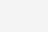# 以太坊智能合约漏洞实战详解：整数溢出攻击

0x01 溢出攻击事件

2018年4月22日，黑客对BEC智能合约发起攻击，凭空取出

``57,896,044,618,658,100,000,000,000,000,000,000,000,000,000,000,000,000,000,000.792003956564819968``

2018年4月25日，SMT项目方发现其交易存在异常，黑客利用其函数漏洞创造了

``65,133,050,195,990,400,000,000,000,000,000,000,000,000,000,000,000,000,000,000 + 50,659,039,041,325,800,000,000,000,000,000,000,000,000,000,000,000,000,000,000``

2018年12月27日，以太坊智能合约Fountain(FNT)出现整数溢出漏洞，黑客利用其函数漏洞创造了

``2 + 115792089237316195423570985008687907853269984665640564039457584007913129639935``

0x02 整数溢出简介

• 乘法溢出
• 加法溢出
• 减法溢出8 位无符整数 255 在内存中占据了 8bit 位置，若再加上 1 整体会因为进位而导致整体翻转为 0，最后导致原有的 8bit 表示的整数变为 0。

``````pragma solidity ^0.4.25;
​
contract POC{
//加法溢出
//如果uint256 类型的变量达到了它的最大值(2**256 - 1)，如果在加上一个大于0的值便会变成0
function add_overflow() returns (uint256 _overflow) {
uint256 max = 2**256 - 1;
return max + 1;
}
​
​
//减法溢出
//如果uint256 类型的变量达到了它的最小值(0)，如果在减去一个小于0的值便会变成2**256-1(uin256类型的最大值)
function sub_underflow() returns (uint256 _underflow) {
uint256 min = 0;
return min - 1;
}

//乘法溢出
//如果uint256 类型的变量超过了它的最大值(2**256 - 1)，最后它的值就会回绕变成0
function mul_overflow() returns (uint256 _underflow) {
uint256 mul = 2**255;
return mul * 2;
}
}````````````pragma solidity ^0.4.25;
​
library SafeMath {
function mul(uint256 a, uint256 b) internal constant returns (uint256) {
uint256 c = a * b;
assert(a == 0 || c / a == b);
return c;
}
​
function div(uint256 a, uint256 b) internal constant returns (uint256) {
uint256 c = a / b;
return c;
}
​
function sub(uint256 a, uint256 b) internal constant returns (uint256) {
assert(b <= a);
return a - b;
}
​
function add(uint256 a, uint256 b) internal constant returns (uint256) {
uint256 c = a + b;
assert(c >= a);
return c;
}
}
​
contract POC{
using SafeMath for uint256;

//加法溢出
//如果uint256 类型的变量达到了它的最大值(2**256 - 1)，如果在加上一个大于0的值便会变成0
function add_overflow() returns (uint256 _overflow) {
uint256 max = 2**256 - 1;
}
​
​
//减法溢出
//如果uint256 类型的变量达到了它的最小值(0)，如果在减去一个小于0的值便会变成2**256-1(uin256类型的最大值)
function sub_underflow() returns (uint256 _underflow) {
uint256 min = 0;
return min.sub(1);
}

//乘法溢出
//如果uint256 类型的变量超过了它的最大值(2**256 - 1)，最后它的值就会回绕变成0
function mul_overflow() returns (uint256 _underflow) {
uint256 mul = 2**255;
return mul.mul(2);
}
}``````0x03 案例分析

BEC合约地址：0xC5d105E63711398aF9bbff092d4B6769C82F793D

``````function batchTransfer(address[] _receivers, uint256 _value) public whenNotPaused returns (bool) {
uint256 amount = uint256(cnt) * _value; //溢出点，这里存在整数溢出
require(cnt > 0 && cnt <= 20);
require(_value > 0 && balances[msg.sender] >= amount);
​
balances[msg.sender] = balances[msg.sender].sub(amount);
for (uint i = 0; i < cnt; i++) {
}
return true;
}``````BEC合约地址：0x55F93985431Fc9304077687a35A1BA103dC1e081

``````function transferProxy(address _from, address _to, uint256 _value, uint256 _feeSmt,
uint8 _v,bytes32 _r, bytes32 _s) public transferAllowed(_from) returns (bool){
​
if(balances[_from] < _feeSmt + _value) revert(); //溢出点，这里存在整数溢出
​
uint256 nonce = nonces[_from];
bytes32 h = keccak256(_from,_to,_value,_feeSmt,nonce);
if(_from != ecrecover(h,_v,_r,_s)) revert();
​
if(balances[_to] + _value < balances[_to]
|| balances[msg.sender] + _feeSmt < balances[msg.sender]) revert();
balances[_to] += _value;
Transfer(_from, _to, _value);
​
balances[msg.sender] += _feeSmt;
Transfer(_from, msg.sender, _feeSmt);
​
balances[_from] -= _value + _feeSmt;
nonces[_from] = nonce + 1;
return true;
}``````

`````` 8fffffffffffffffffffffffffffffffffffffffffffffffffffffffffffffff _value
+
7000000000000000000000000000000000000000000000000000000000000001 _feeSmt
=
10000000000000000000000000000000000000000000000000000000000000000``````FNT合约地址：0x82Cf44bE0768A3600c4BDeA58607783A3A7c51AE

``````function batchTransfers (address[] receivers, uint256[] amounts) public whenRunning returns (bool) {
​
uint256 totalAmount = 0;
uint i;
for (i = 0; i < receiveLength; i ++) {
if (r == address(0) || r == owner) continue;
totalAmount += amounts[i]; ////溢出点，这里存在整数溢出，计算需要转出的全部数额
}
require(totalAmount > 0);
require(canPay(msg.sender, totalAmount)); //这里判断msg.sender的余额大于totalAmount
​
wallets[msg.sender] -= totalAmount;
uint256 amount;
for (i = 0; i < receiveLength; i++) {
if (r == address(0) || r == owner) continue;
amount = amounts[i];
if (amount == 0) continue;
emit Transfer(msg.sender, r, amount); //逐一转账
}
return true;
}``````

``````2                                                                               amount
+
115792089237316195423570985008687907853269984665640564039457584007913129639935  amount
=
1                                                                             totalAmount``````0x04 总结

(0)